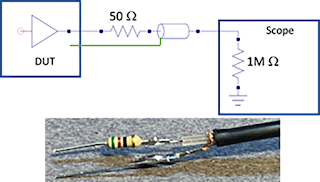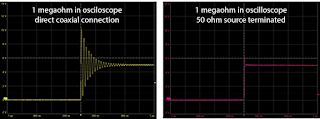## You need to test, we're here to help.

You need to test, we're here to help.

## 22 June 2020

### Build Your Own Low-Cost Power Rail ProbeFigure 1: The source series termination method is a low-cost alternative for probing low-impedance, fast-switching sources.

In an earlier post, we discussed the limitations of  Using 50-Ohm Coax from DUT to Oscilloscope  with low-voltage, high-bandwidth signals, like power rails. In this post, we’ll explain how to build your own, low-cost power rail probe to serve the purpose.

The source series termination method is a good alternative for probing a low-impedance, fast-switching source, comprising a 50-ohm resistor in series between the DUT and the coaxial-cable connection. The coaxial cable is then connected to the oscilloscope’s analog input set for 1 megaohm termination. An equivalent circuit model and a simple implementation appears in Figure 1.

When measuring most high-bandwidth signals, 50 ohm is the recommended input impedance to the oscilloscope. This does not hold true, however, for power rails. For one, with 50-ohm input impedance, the maximum voltage one can probe is typically around 5 V. Higher voltages will dissipate too much power in the 50-ohm resistor, and the oscilloscope may suffer damage. For another, using 50-ohm input impedance will introduce a DC load to the DUT.Figure 2. Equivalent circuit for a direct coax connection to a 1 megaohm oscilloscope input termination.
Thus, we must use the oscilloscope’s 1 megaohm input. On one hand, this enables measurement of a voltage range of ±40 V with a negligible DC current draw. On the other, when probing a low-impedance source via a direct coax connection, the oscilloscope’s 1 megaohm input termination will generate large reflections from fast transient edges. The equivalent circuit is shown in Figure 2.

The answer to this problem: add a 50-ohm source series termination at the end of the coaxial cable. As a result, half of the source voltage is initially launched into the coax cable, which reaches the oscilloscope’s 1 megaohm input termination and reflects back. The initial voltage measured by the oscilloscope is 2x the launched voltage, which is exactly the voltage of the source.Figure 3. Startup of 5 V power supply with direct coax connection to oscilloscope 1 megaohm input (left), and with a 50-ohm source series termination added (right).
When the reflected signal makes its way to the source, it sees the 50-ohm resistor in series with the low impedance of the source. As long as the source impedance is less than 5 ohm, there is virtually no reflection and the reflections are terminated. Figure 3 shows an example of a 5 V switched mode power supply input with a direct coaxial cable connection and with a 50-ohm source series termination. This simple approach of adding a 50-ohm source series resistance is a low-cost alternative to probing power rails at high bandwidth. It is an alternative to using a 10x probe, with the advantage of being a 1x probe that does not attenuate the signal.

Of course, a far better solution, if your budget permits, is the RP4030 Active Voltage Rail Probe, which is designed specifically to probe a 50-ohm DC voltage/power rail while coupled to the 50 ohm oscilloscope input for the lowest noise and minimal DUT loading.

Earlier posts: